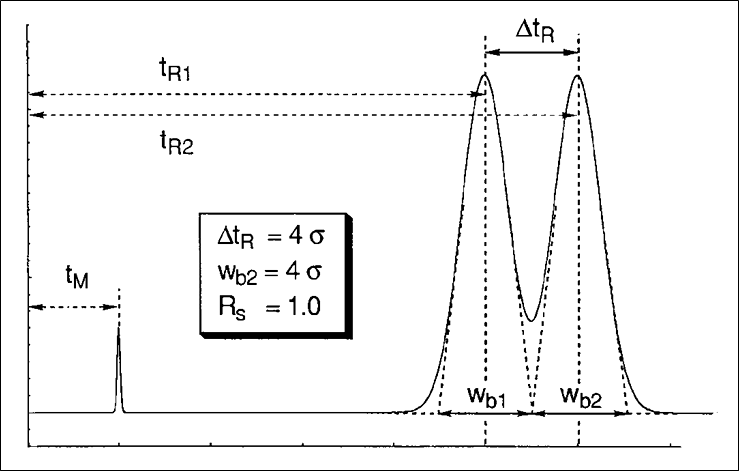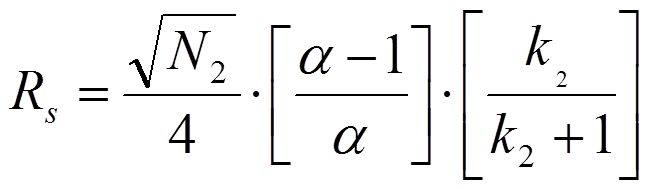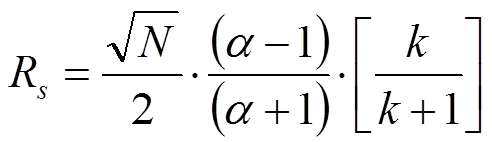NOTE: Figure and equation numbers are shown by moving over the figure or the equation. They are not shown separately.

On this page, I want to deal with the different formulations for resolution used in HPLC and explain the subtle differences between the formulas that are used. These explanations have been taken from an article by A.S. Said (J. High Resolution Chromatograpy &Chromatography Communciations, (1979) 193).Figure 1 shows the essential parameters to measure resolution in HPLC. The formula for resolution is given below.resulting in resolution = 1 after substituting the values given in the figure on the left.

The issue described here is that in simplification of equation 1.1, different assumptions can be made which lead to different expressions for resolution in chromatographic parameters.

In a first approach, issued by Purnell (J. Chem. Soc., (1960) 1268), it is assumed that the width of the first peak equals the width of the second peak. After some algebraic manipulations, one arrives at equation 1.2:In equation 1.2. N2 is the plate number of the second peak, k2 is the retention factor of the second peak and alpha is the selectivity factor defined as the ratio of k2 and k1. However, when one assumes that the peak width of the second peak equals the width of the first peak one arrives at equation 1.3 which is known as the Knox equation for resolution:It is imperative to realize the difference between these two equations for the resolution of chromatographic peaks. Many mistakes are easily observer especially in non-peer reviewed journals and in promotional literature from HPLC column manufacturers. In most cases the indices are omitted. This implies that the average plate number and average of k1 and k2 are used. In fact however, in case the average values of N and k of the two peaks under review according to Said (JHRCCC, (1979) 193) the resolution equation changes to:More details can be found in the publication by Prof. Pat Sandra, J. High Resolution Chromatography, 12, 82 (1989).

In a very detailed and informative review, Prof. Joe Foley has expanded this topic further and has provided additional accurate formulations of the resolution equation (Table 1, eqn. 9-11 in J.P. Foley, Analyst 116, 1275 (1991)). Foley has emphasized the assumptions that lay at base of the different resolution equations. He eventually proposed three additional equations for the resolution of chromatographic bands elutig from the column.